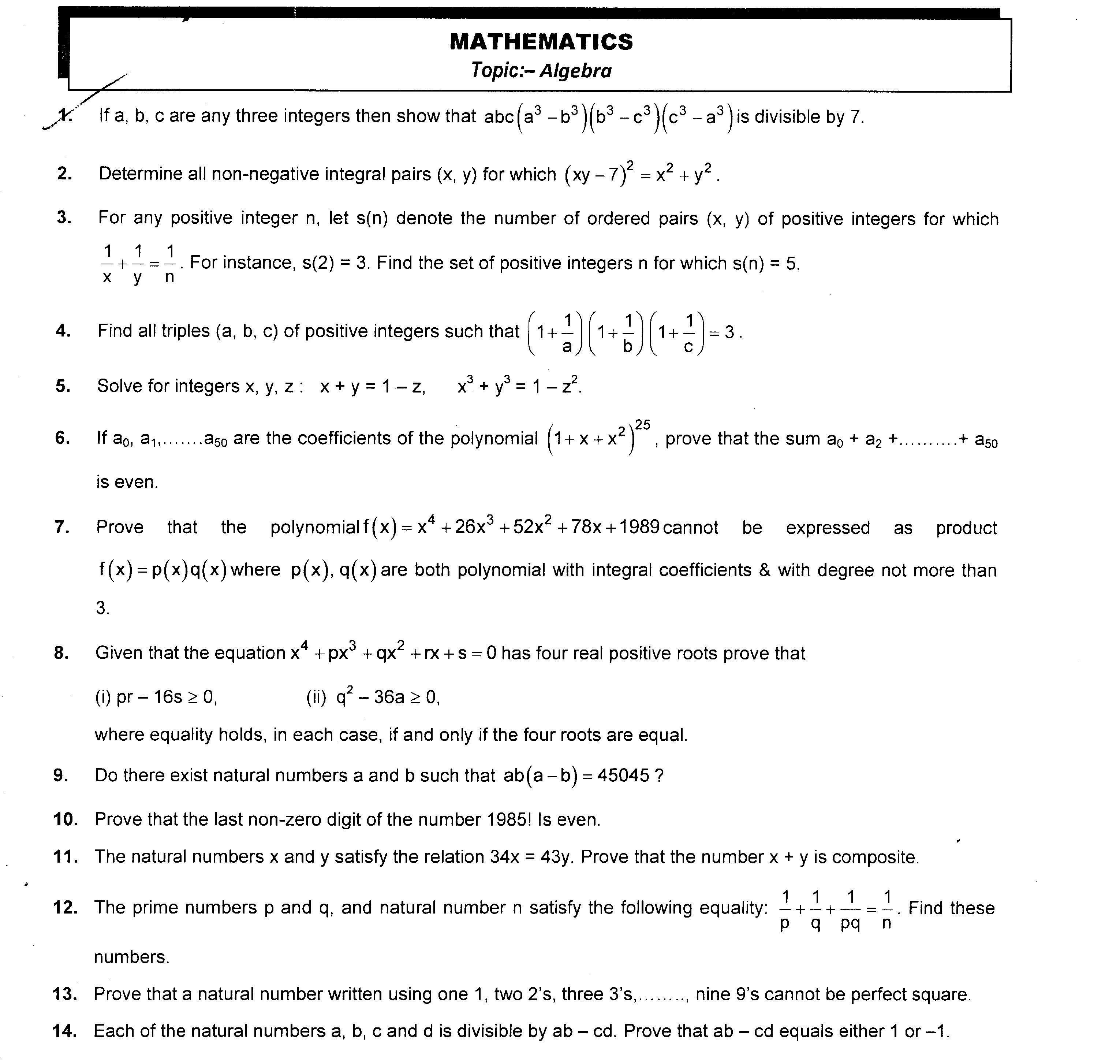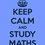# Maths RMO Problems(1)........

Hey friends.

I mean Brilliantians I am back with some amazing problems which are generally asked in the RMO-INMO level examination .I am sharing the image of the paper containing the questions.

Please try and if possible send the solutions

.Also it would be great if you all participate in sharing the questions from your own. I would also be sharing problems based on NSEP level. ThanksDont forget to share and like this.............Note by Abhisek Mohanty
3 years, 3 months ago

This discussion board is a place to discuss our Daily Challenges and the math and science related to those challenges. Explanations are more than just a solution — they should explain the steps and thinking strategies that you used to obtain the solution. Comments should further the discussion of math and science.

When posting on Brilliant:

• Use the emojis to react to an explanation, whether you're congratulating a job well done , or just really confused .
• Ask specific questions about the challenge or the steps in somebody's explanation. Well-posed questions can add a lot to the discussion, but posting "I don't understand!" doesn't help anyone.
• Try to contribute something new to the discussion, whether it is an extension, generalization or other idea related to the challenge.

MarkdownAppears as
*italics* or _italics_ italics
**bold** or __bold__ bold
- bulleted- list
• bulleted
• list
1. numbered2. list
1. numbered
2. list
Note: you must add a full line of space before and after lists for them to show up correctly
paragraph 1paragraph 2

paragraph 1

paragraph 2

[example link](https://brilliant.org)example link
> This is a quote
This is a quote
    # I indented these lines
# 4 spaces, and now they show
# up as a code block.

print "hello world"
# I indented these lines
# 4 spaces, and now they show
# up as a code block.

print "hello world"
MathAppears as
Remember to wrap math in $$ ... $$ or $ ... $ to ensure proper formatting.
2 \times 3 $2 \times 3$
2^{34} $2^{34}$
a_{i-1} $a_{i-1}$
\frac{2}{3} $\frac{2}{3}$
\sqrt{2} $\sqrt{2}$
\sum_{i=1}^3 $\sum_{i=1}^3$
\sin \theta $\sin \theta$
\boxed{123} $\boxed{123}$

Sort by:

Question 2

$(xy-7)^2=x^2+y^2\Rightarrow x^2y^2-14xy+49=x^2+y^2 \\ x^2y^2-12xy+36+13=x^2+y^2+2xy \\ (x+y+xy-6)(x+y-xy+6)=13=13×1=1×13=-13×-1=-1×-13 \\ (x,y)=(3,4),(4,3),(0,7),(7,0)$

- 3 years, 3 months ago

Nice solution................upvoted....

- 3 years, 3 months ago

Question 1 \begin{aligned} x &\equiv 0,1,2,3,4,5,6 \pmod{7}\\ x^3 &\equiv 0,1,-1\pmod{7} \end{aligned}

Assume that either of $a,b,c$ is a multiple of 7.

Then obviously $abc(a^3-b^3)(b^3-c^3)(c^3-a^3)$ is a multiple of 7.

So now WLOG assume that $a,b,c\not\equiv 0\pmod{7}$.Then $a^3,b^3,c^3\equiv 1,-1\pmod{7}$.There are $2\times 2\times 2=8$ different possible cases corresponding to the different values of $a^3,b^3$ and $c^3$ modulo 7,which are: $\begin{array}{c|c|c|c} \text{Values modulo 7} & a^3 & b^3 & c^3 \\ \hline \text{Case 1} & 1 & 1 & 1 \\ \hline \text{Case 2} & -1 & -1 & -1 \\ \hline \text{Case 3} & 1 & -1 & -1 \\ \hline \text{Case 4} & -1 & 1 & -1 \\ \hline \text{Case 5} & -1 & -1 & 1 \\ \hline \text{Case 6} & -1 & 1 & 1 \\ \hline \text{Case 7} & 1 & -1 & 1 \\ \hline \text{Case 8} & 1 & 1 & -1 \end{array}$

Observe that,because of the symmetry of the expression,Cases 3,4,5 and Cases 6,7,8 are equivalent.Therefore,we only need to check Case 1,2,3 and 6.Simply evaluate the cases to get that $abc(a^3-b^3)(b^3-c^3)(c^3-a^3)\equiv 0\pmod{7}\;\forall \;a,b,c\in \mathbb{Z}$

- 3 years, 3 months ago

Nice solution bro..........upvoted

- 3 years, 3 months ago

vmc questions

- 3 years, 3 months ago

Question 8(i)

Let the roots be $a,b,c,d$.(Note that the roots are positive).Then: \begin{aligned} p&=-(a+b+c+d)\\ q&=ab+ac+ad+bc+bd+cd\\ r&=-(abc+abd+acd+bcd)\\ s&=abcd \end{aligned} $pr-16s\geq 0\implies (a+b+c+d)(abc+abd+acd+bcd)\geq 16abcd$ which follows by applying AM-GM on each term.

I couldn't understand;what does the variable "a" denote in Q 8(ii)?

- 3 years, 3 months ago

Question 9 We shall analyze 2 cases. Case 1 Either of $a,b$ is even.

WLOG let $a$ be even.Then $ab(a-b)=45045$ is even.But 45045 is odd,contradiction. Hence no solutions exist in this case.

Case 2 Both $a,b$ are odd.

Then $a-b$ is even.Therefore $ab(a-b)=45045$ is even,contradiction.

Hence no solutions exist.

- 3 years, 3 months ago

- 3 years, 3 months ago

question 11 given 34x=43y =>34x+43x=43(y+x) =>77x=43(x+y) now 43 does not divide 77 hence x+y contains 77 i.e-11*7 hence x+y is not prime.

- 3 years, 3 months ago

That does not exclude the possibility of x+y being odd but not prime

- 3 years, 3 months ago

Can you please explain me what u are trying to say?

- 3 years, 3 months ago

Can anyone recommend me some good books for INMO and and other maths olympiad??????????

- 3 years, 3 months ago

Q13 The sum of the digits of any number formed using the given conditions is 1 + 4 + 9+ . . . . + 81 = 285 = 3(95) which implies the number is divisible by 3 but not by 3 squared which is 9. Therefore any number formed using the given conditions is not a perfect square

- 3 years, 1 month ago